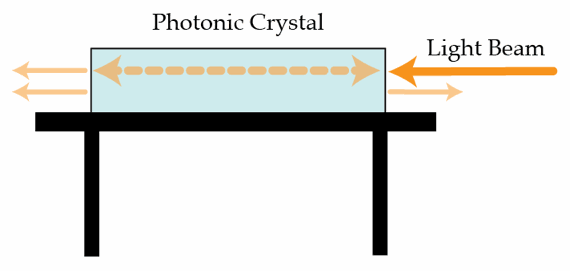# One-dimensional photonic crystal

Photonic crystals are materials which have dielectric constant which varies periodically, thus creating many interesting phenomena. One-dimensional photonic crystals are made from parallel layers with varying dielectric constants. We can analyze the unit cell of such crystals by examining the effect a single dielectric crystal has on a beam of light.

Suppose light enters the glass crystal in the image below from the right. Some percentage of the light will exit from the left and some from the right. The transmission coefficient at the air/glass interface is $t = 0.7$, and is the same in both directions. How much of the light in % exits the crystal on the left?×